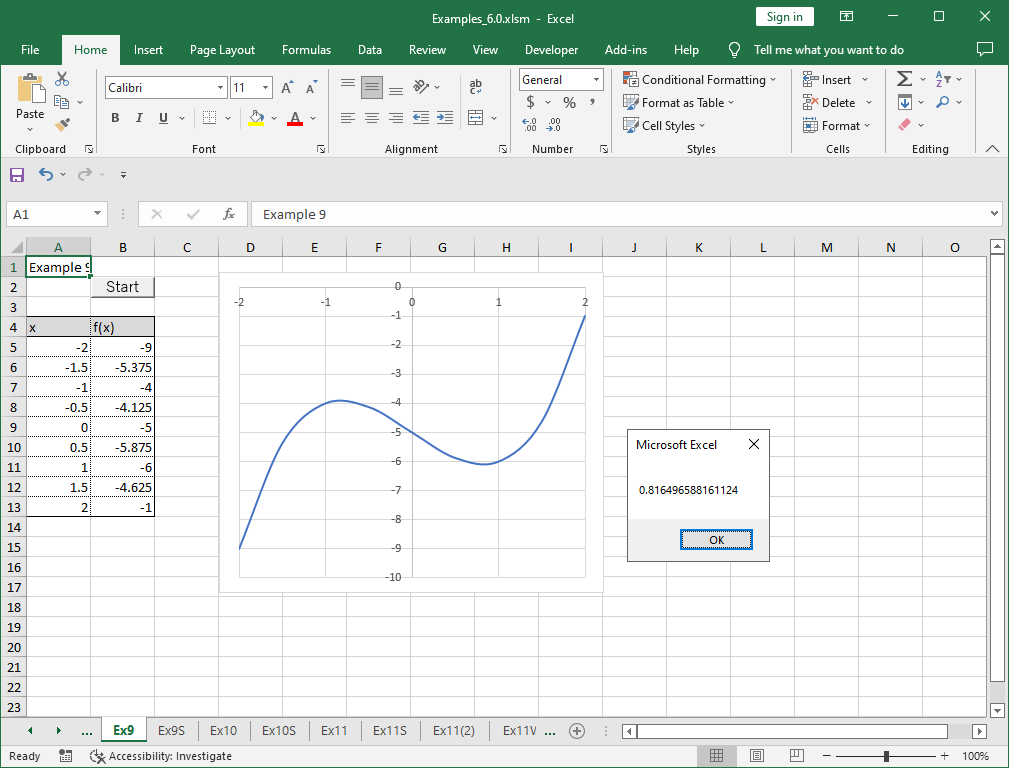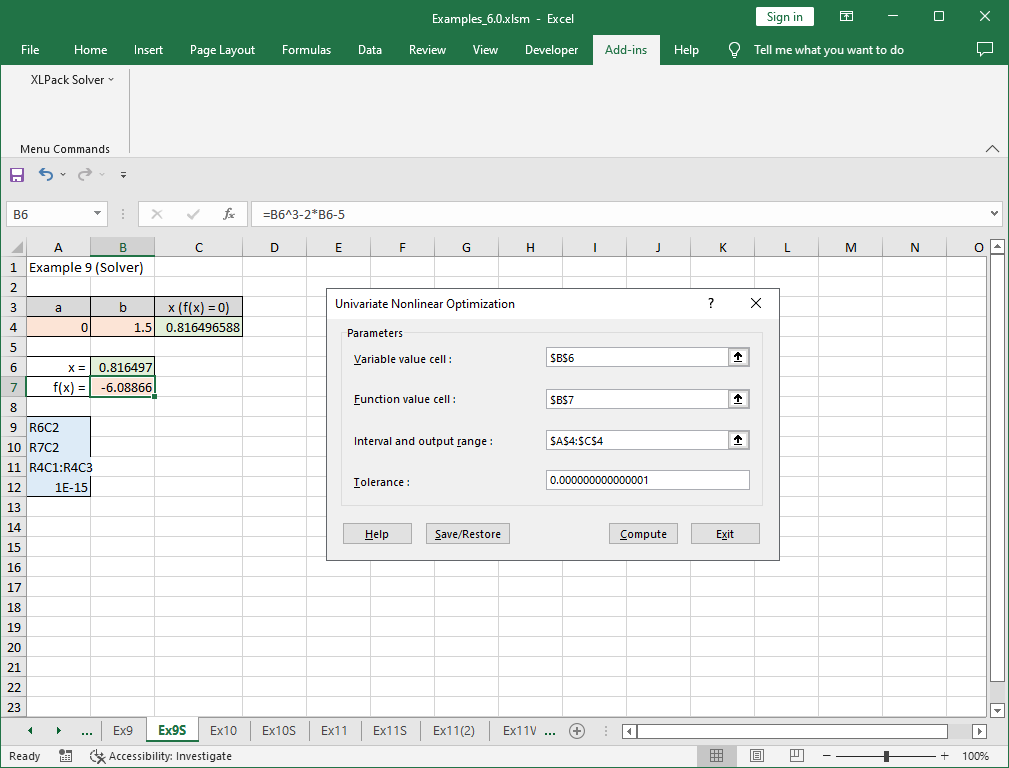# 9. Nonlinear optimization of univariate function

Note – The example worksheets are available for download. See 1. How to obtain and use example worksheets.

Example of solving a nonlinear optimization of univariate function, i.e. finding a minimum point of general one-dimensional nonlinear function by XLPack is shown below.

### Example

Let’s obtain the minimum point of the following equation near x = 1.

```
f(x) = x3 - 2x - 5 = 0

```

As shown in the figure below, there is no global minimum of f(x) (diverging for -∞). However, there is a local minimum near x=1.### Solving problem with VBA program (1)

The following is an example code using VBA library function Dfmin.

``````Function F(X As Double) As Double
F = X ^ 3 - 2 * X - 5
End Function

Sub Start()
Dim Ax As Double, Bx As Double, Tol As Double, X As Double
'--- Input data
Ax = 0
Bx = 1.5
Tol = 0.000000000000001 '1e-15
'--- Compute min. point of equation
Call Dfmin(Ax, Bx, AddressOf F, Tol, X)
'--- Output min. point
MsgBox X
End Sub``````

The function Dfmin finds the minimum point in the interval between given Ax and Bx. Only one minimum is assumed in the interval. In this example, the local minimum exists near x = 1. Then, Ax = 0 and Bx = 1.5 are set in the program.

The result X = 0.81650 is obtained by running this program.### Solving problem with VBA program (2)

The following is an example code using the reverse communication version VBA library subroutine Dfmin_r.

``````Sub Start()
Dim Ax As Double, Bx As Double, Tol As Double, X As Double
Dim XX As Double, YY As Double, IRev As Long
'--- Input data
Ax = 0
Bx = 1.5
Tol = 0.000000000000001 '1e-15
'--- Compute min. point of equation
IRev = 0
Do
Call Dfmin_r(Ax, Bx, Tol, XX, YY, IRev)
If IRev <> 0 Then YY = XX ^ 3 - 2 * XX - 5
Loop While IRev <> 0
X = XX
'--- Output min. point
MsgBox X
End Sub``````

Instead of defining the object function as the external VBA function, when returned with IRev = 1, you need to compute the object function value at XX, enter it in YY and call Dfmin_r again. For further details of reverse communication version, see here.

The same result with above is obtained by this program.

### Solving problem with Solver

“Univariate Nonlinear Optimization” of XLPack solver add-in can be used to solve this problem. The formula (=B6^3-2*B6-5) is entered in cell B7.Please refer to here for how to use solver.